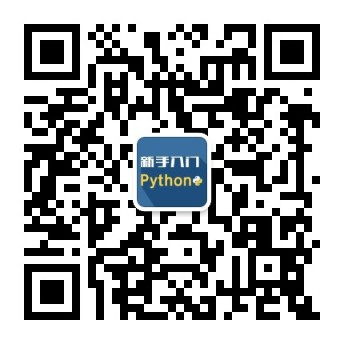2023
03-07

# python入门小程序教程如何编写（python小程序开发教程步骤）Python环境中，输入以下代码：

print("Hello World")

plaintext

Copy code

num1 = float(input("输入第一个数字："))

num2 = float(input("输入第二个数字："))

print("请选择要进行的操作：")

print("1.加法")

print("2.减法")

print("3.乘法")

print("4.除法")

choice = input("请输入您的选择（1/2/3/4）：")

if choice == '1':

print(num1, "+", num2, "=", num1+num2)

elif choice == '2':

print(num1, "-", num2, "=", num1-num2)

elif choice == '3':

print(num1, "*", num2, "=", num1*num2)

elif choice == '4':

print(num1, "/", num2, "=", num1/num2)

else:

print("输入的选择无效")

4. 编写猜数字游戏

plaintext

Copy code

import random

number = random.randint(1, 100)

while True:

guess = int(input("猜一个1~100之间的数字："))

if guess == number:

print("恭喜您，猜对了！")

break

elif guess < number:

print("猜的数字太小了，再试试吧！")

else:

print("猜的数字太大了，再试试吧！")